## 1. Background

Do you often need to merge Excel worksheets with same headers for further analysis? If the answer is yes, then I figured that you find it an annoyance to do the combination, particularly when there are many worksheets or files. Even Excel VBA often fails if there is a huge amount of data. In this case, a professional stand-alone data computing tool will be helpful. esProc is one of its kind, an outstanding one. There will be no need to write complex and inefficient VBA code, but a few lines of SPL (abbreviation of Structured Process Language with innovative syntax that esProc uses) code will suffice instead. With esProc at hand, merging Excel worksheets will no longer be a nightmare.

## 2. Basic merges

#### A．Merge multiple sheets of one Excel file

Below is an Excel file containing sales data. There are 3 worksheets of same structure in the file.

january_2013:

february_2013:march_2013: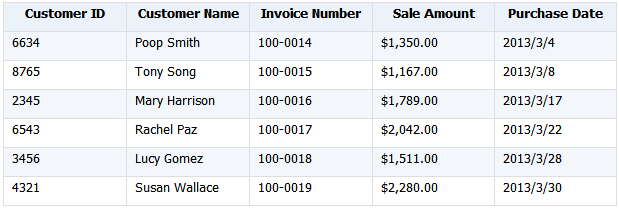The merging requires getting two fields, Customer Name & Sales Amount, from each worksheet. Below is the final merging result: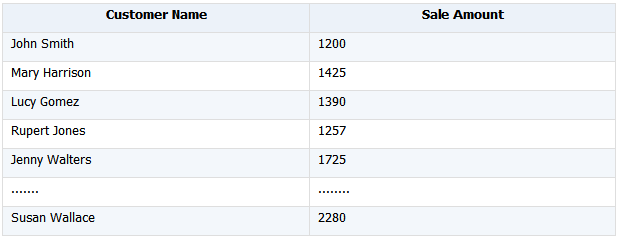esProc SPL script:
A
1 =file("D:/sales_2013.xlsx").xlsopen()
2 =A1.conj(A1.xlsimport@t('Customer Name','Sale Amount';~.stname))
3 >file("D:/result_2013.xlsx"). xlsexport@t(A2;"merge_sheets")

Explanation:
A1: Open the Excel file and generate a sequence of 3 worksheets.
A2: conj() function traverses the member worksheets in A1’s sequence to import the specified fields ‘Customer Name’ and ‘Sale Amount’ and merges them. xlsimport() function imports the fields, where a semicolon follows the last field and parameter ~.stname represents the current worksheet. @t option specifies that the first row in each worksheet will be the field names. The function is nested within conj() function’s loop traversal to import data from every worksheet.
A3: Save A2’s table sequence as a new worksheet in the original Excel file with the name “merge_sheets”. @t option is used to make the first row the column headers.

Only three lines of code, the script is short, concise yet clear and easy to understand.

#### B．Merge multiple sheets from different Excel files

Here are several Excel files in which every worksheet containing a year’s sales data has the same structure as the worksheets in the previous instance:

esProc SPL script:

A B
1 for directory@p("d:/excel/*.xlsx") =file(A1).xlsopen()
2 =B1.conj(B1.xlsimport@t('Customer Name','Sale Amount','Purchase Date';~.stname))
3 =@|B2
4 > file("d:/result.xlsx"). xlsexport@t(B3;"merge_data")

The desired merging effect:Explanation:
A1: The for loop traverses every Excel file under the given directory to perform a series of operations over every worksheet in B1~B3.
B1: Open an Excel file and generate a sequence.
B2: Import the specified fields, ‘Customer Name’, ‘Sale Amount’ and ‘Purchase Date’, from every worksheet of the current file and merge them. This is similar to the operations in A2 in the previous instance.
B3: Combine B2’s table sequence with the current value of B3.
A4: Save B3’s table sequence as a worksheet, named merge_data, in file result.xlsx.

The program achieves multiple Excel file merging with only two rounds loop. The outer loop traverses each of the Excel files under the given directory while the inner loop B1.conj() function merges data from every worksheet in an Excel file.

#### C．File merging with big result set

Both A2 in the first instance and B3 in the second instance receive all merged data in the memory and then write it out at once. But a big merged result set can use up too much memory space or the available memory space won’t be enough to hold the data. In that case, stream-style appending can be used to generate a big file.

esProc SPL script:
A B
1 =file("D:/out.xlsx")
2 for directory@p("d:/excel/*.xlsx") =file(A2).xlsopen()
3 =if(A1.exists(),B2.xlsimport@t(),B2.xlsimport())
4 >A1.xlsexport@s(B3;"merger")

The desired merging effect:

Explanation:
A1: Open a specified file into which data will be output.
A2: Traverse all to-be-merged Excel files under the given directory.
B2: Open a to-be-merged Excel file.
B3: If the file into which data will be output exists, import all rows, including the column headers, of a worksheet; if the file exists, use @t option to make the first row the column headers and import data from the second row.
B4: Import data in a stream style in B3 and append it to the worksheet named merger in A1’s specified file.

The stream-style importing and appending is suited to combine many small excel files into a big one.

## 3. Grouping & aggregation

We continue to use the previous Excel file containing the sales data maker further analysis.

#### A. Group data by one or more fields

Here’s an eProc SPL script for grouping data by one or multiple fields:
A
1 =file("D:/sales_2013.xlsx").xlsopen()
2 =A1.conj(A1.xlsimport@t(;~.stname))
3 =A2.groups('Customer ID';sum('Sale Amount'):Total,avg('Sale Amount'):Average)
4 =A2.groups('Customer ID','Purchase Date';sum('Sale Amount'):Total)

A3’s grouping result: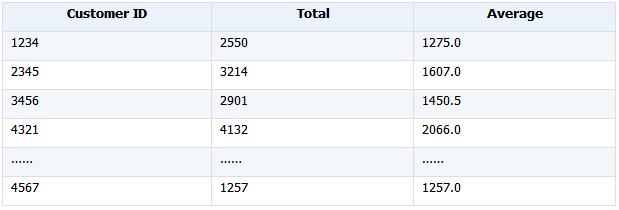A4’s grouping result:

Explanation:
A1: Open the specified Excel file.
A2: Import all data from the worksheets in the file and perform merging.
A3: Group the merged data by ‘Customer ID’ and calculate total and average for each group.
A4: Group the merged data by ‘Customer ID’ and ‘Purchase Date’, and calculate total for each group.

#### B. Group data in a certain order

esProc supports comparing neighboring records during grouping and aggregation. If the data is already ordered, then a re-sorting will be unnecessary. Now suppose the original data is ordered by date, but we need to summarize data by month.

esProc SPL script:
A B
1 for directory@p("d:/excel/*.xlsx") =file(A1).xlsopen()
2 =B1.conj(B1.xlsimport@t(;~.stname))
3 =@|B2
4 =B3.derive(year('Purchase Date'):Year,month('Purchase Date'):Month)
5 =A4.groups (month('Purchase Date'):Month;sum('Sale Amount'):Total,avg('Sale Amount'):Average)
6 =A4.groups@o (month('Purchase Date'):Month;sum('Sale Amount'):Total,avg('Sale Amount'):Average)

A5’s grouping result:

A6’s grouping result:

Explanation:
A1~B3: Merge all worksheets in the Excel files of same structure under the specified directory.
A4: Generate a new table sequence base on B3’s table sequence by splitting the Purchase Date field into a new Year field and a new Month field.
A5: groups() function groups data by month and calculates total and average for each group.
A6: groups@o groups data in each year by month, calculates total and average for each group, and, with @o present, merges the summarized results.

A4 contains detailed sales records; A5 summarizes data only by month, ignoring the year; A6 summarizes data by both year and month. The three cells shows summarized data of different levels.

#### C. Group data by segment

This type of grouping divides data into multiple segments according to specified conditions and summarizes each group of data.

esProc SPL script:
A B
1 for directory@p("d:/excel/*.xlsx") =file(A1).xlsopen()
2 =B1.conj(B1.xlsimport@t(;~.stname))
3 =@|B2
4 =B3.groups(if ('Sale Amount'<1000,"1::<1000",
if ('Sale Amount'<1500,"2::1000~~1500",
if ('Sale Amount'<2000,"3::1500~~2000",
if ('Sale Amount'<2500,"4::2000~~2500",
"5::>=2500")))):Segment; count(1):Number,sum('Sale Amount'):Total)

Grouping result: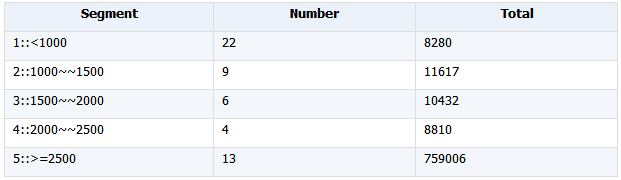Explanation:
About the code block A1~B3, refer to the explanations of previous instances.
A4: It defines 5 intervals for the value of ‘Sale Amount’ field and calculates number and total for within each range.

But the above script is still not convenient-to-use enough. When we want to change the way of dividing the data, we need to modify the parameter expressions in groups() function. This is a little complicated. Another function, pseg(), helps to achieve an easier modification. Here’s the SPL code:
A B
1 [0,1000,1500,2000,2500]
2 for directory@p("d:/excel/*.xlsx") =file(A1).xlsopen()
3 =B1.conj(B1.xlsimport@t(;~.stname))
4 =@|B2
5 =B4.groups(A1.pseg(~.'Sale Amount'):Segment; count(1):Number,sum('Sale Amount'):Total)

We can also group data by multiple fields according to a set of conditions and then summarize each group. For example, we divide the scores of subjects into 5 grades – Excellent, Good, Satisfactory, Poor, Passing, and summarize each group. For more uses of groups() function, refer to esProc Function Reference.

#### D. Group big data

In the above instances, the Excel file(s) is/are fairly small to be imported into the memory at a time. If the total data amount is huge, trying to retrieve all data into the memory in either esProc or VBA could fail. An alternative is the stream retrieval that reads a part of data into the memory and summarizes it at one time.

esProc SPL script:
A B
1 =file("d:/tdata.xlsx").xlsopen@r()
2 for A1.count() =A1.xlsimport@ct(;A1(A2). stname)
3 =@|B2
4 = B3.conjx() =A4.groups('Customer ID';sum('Sale Amount'):SaleTotal)
5 >file(“d:/out.xlsx”).exportxls@bt(B4;"Customer&Sales")

The result of filtering and then grouping data:Explanation:
A1: Use @r option to open an Excel file in a stream style.
A2: Traverse every worksheet in the Excel file.
B2: Use @c option to import data with cursor.
B3: Union B2’s cursor into B3.
A4: Union members of B3’s cursor sequence into a new cursor.
B4: Group A4’s sequence by Customer ID and calculate Sale Amount for each group.
A5: Export and save B4’s result set into an Excel file.

Each time, the stream retrieval reads a part of data with cursor and groups and summarizes it.

## 4.Deduplication

Duplicate data affects the analysis results. As duplication is common in real-world cases, let’s look at how to remove duplicates in esProc SPL.

#### A. Via primary key

Set ‘Invoice Number’ as the primary key of sales_2013 to remove duplicates according to it.
A
1 =file("d:/sales_2013.xlsx").xlsopen()
2 =A1.conj(A1.xlsimport@t('Customer Name', 'Invoice Number', 'Sale Amount';~. stname))
3 =A2.group@1('Invoice Number')
4 >file(“d:/out.xlsx”). xlsexport@t(A3;"result")

Concatenated deduplicated data: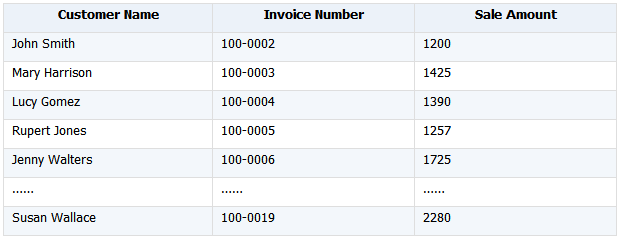Explanation:
A1: Open a certain Excel file.
A2: Import specified columns from every worksheet.
A3: Group A2’s table sequence by primary key ‘Invoice Number’ and deduplicate each group.
A4: Export and save A3’s result set into an Excel file.

Data is distinct in each worksheet, but probably not once the worksheets are concatenated. Primary key is one of the ways of deduplicating data.

#### B. Via a certain field

Deduplicate data in sales_2013 according to a certain field to look through employee records of different names.
A
1 =file("d:/sales_2013.xlsx").xlsopen()
2 =A1.conj(A1.xlsimport@t('Customer ID', 'Customer Name';~. stname))
3 =A2.id('Customer Name')
4 =A2.group@1('Customer Name')
5 >file("d:/out.xlsx"). xlsexport@t(A4;"result")

Explanation:
A1: Open a certain Excel file.
A2: Import specified columns from every worksheet.
A3: Get a sequence of distinct names from A2’s table sequence.
A4: Get a table sequence of distinct names from A2’s table sequence.
A5: Export and save data of A4’s table sequence into an Excel file, with the first row as the column headers.

A3’s deduplication result: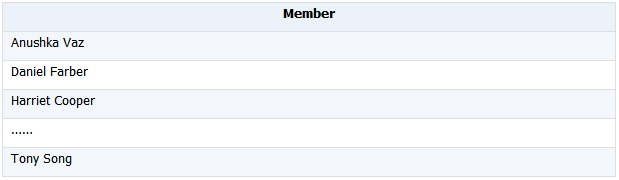A4’s deduplication result:#### C. Via multiple fields

Sometimes we need several other fields, instead of the primary key, to determine whether records are duplicates or not.
A
1 =file("d:/sales_2013.xlsx").importxls@t()
2 =file("d:/sales_2014.xlsx").importxls@t()
3 =[A1,A2].merge('Customer ID', 'Purchase Date')
4 =A3.group@1('Customer ID', 'Purchase Date')
5 >file("d:/out.xlsx"). xlsexport@t(A4;"result")

Explanation:
A1: Import a specified Excel file.
A2: Import another specified Excel file.
A3: Merge A1’s table sequence and A2’s table sequence by fields ‘Customer ID’ and ‘Purchase Date’ and return a new table sequence.
A4: Group and deduplicate A3’s table sequence by ‘Customer ID’ and ‘Purchase Date’.
A5: Export and save 43’s result set into an Excel file.

We can perform the merge and grouping and deduplication by more fields as needed.

#### D. Deduplicate records

Records in each file are distinct, but the concatenated file could contain duplicate records.
A B
1 =file("d:/sales_2013.xlsx").importxls@t() =A1.group@1('Invoice Number')
2 =file("d:/sales_2014.xlsx").importxls@t() =A2.group@1('Invoice Number')
3 =[B1,B2].merge@u() =A3.count()

Explanation:
A1: Import a specified Excel file.
B1: Deduplicate A1’s table sequence by ‘Invoice Number’.
A2&B2: Same as A1&B1.
A3: Merge B1’s table sequence and B2’s table sequence, deduplicate data, and return a table sequence. @u option is used to merge multiple table sequences in order, remove duplicates, and generate a new table sequence.
B3: Count the records in the merged table sequence. merge@u() function merges multiple ordered, deduplicated table sequences.

In the above, we explain how to handle merging, grouping and summarization, and deduplication over same-structure Excel files in esProc SPL. For Excel files of different structures, we just need to read to-be-merged fields as a sequence object and then process it in same ways. It is similar to handle other types of text files.

# 1. Aligned grouping

Example 1: List the number of countries whose official languages are respectively Chinese, English and French.

MySQL8:
```with t(name,ord) as (select 'Chinese',1 ```
```      union all select 'English',2 ```
```      union all select 'French',3) ```
```select t.name, count(countrycode) cnt ```
```from t left join world.countrylanguage s on t.name=s.language ```
```where s.isofficial='T' ```
```group by name,ord ```
```order by ord; ```

Note: The charset the table uses and the session charset should be the same.
(1) show variables like ‘character_set_connection’ View the charset the current session uses;
(2) show create table world.countrylanguage View the table’s charset;
(3) set character_set_connection=[Charset]Update the charset the current session uses

esProc SPL script:
A
1 =connect("mysql")
2 =A1.query@x("select * from world.countrylanguage where isofficial='T' ")
3 [Chinese,English,French]
4 =A2.align@a(A3,Language)
5 =A4.new(A3(#):name, ~.len():cnt)

A1: Connect to the database;
A2: Get all records of official languages;
A3: List three official languages;
A4: Align all records with A3’s members in order by Language;
A5: Create a table sequence consisting of language name and the number of countries that use the language.Example 2: List the number of countries whose official languages are respectively Chinese, English, French and others.

MySQL8:
```with t(name,ord) as (select 'Chinese',1 union all select 'English',2 ```
```    union all select 'French',3 union all select 'Other', 4), ```
```        s(name, cnt) as ( ```
```             select language, count(countrycode) cnt ```
```             from world.countrylanguage s ```
```             where s.isofficial='T' and language in ('Chinese','English','French') ```
```             group by language ```
```    union all ```
```        select 'Other', count(distinct countrycode) cnt ```
```        from world.countrylanguage s ```
```        where isofficial='T' and language not in ('Chinese','English','French') ) ```
``` ```
```select t.name, s.cnt ```
```from t left join s using (name) ```
```order by t.ord; ```

esProc SPL script:
A
1 =connect("mysql")
2 =A1.query@x("select * from world.countrylanguage where isofficial='T' ")
3 [Chinese,English,French,Other]
4 =A2.align@an(A3.to(3),Language)
5 =A4.new(A3(#):name, if(#<=3,~.len(), ~.icount(CountryCode)):cnt)

A4: Align all records with A3.to(3) in order by Language, and append a group containing non-aligned records;
A5: Over the last group, calculate the number of countries with other CountryCodes.# 2. Enum grouping

Example 1: List the number of cities of different types according to the specified order.

MySQL8:
```with t as (select * from world.city where CountryCode='CHN'), ```
```segment(class,start,end) as (select 'tiny', 0, 200000 ```
```    union all select 'small', 200000, 1000000 ```
```    union all select 'medium', 1000000, 2000000 ```
```    union all select 'big', 2000000, 100000000 ```
```) ```

``` select class, count(1) cnt ```
```from segment s join t on t.population>=s.start and t.population<s.end ```
```group by class, start ```
```order by start; ```

esProc SPL script:
A
1 =connect("mysql")
2 =A1.query@x("select * from world.city where CountryCode='CHN' ")
3 =\${string([20,100,200,10000].(~*10000).("?<"/~))}
4 [tiny,small,medium,big]
5 =A2.enum(A3,Population)
6 =A5.new(A4(#):class, ~.len():cnt)

A3: \${…} is the macro replacement, which calculates the expression enclosed by the braces and takes the result as a new expression for recalculation. Here the final result is the sequence [“?<200000”,“?<1000000”,“?<2000000”,“?<100000000”].
A5: Over each record in A2, compare it with A3’s first condition, and add it to the corresponding group if the condition is satisfied.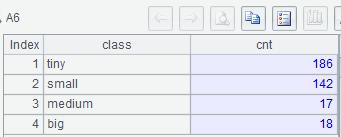Example 2: List the numbers of metropolises in East China, in other areas in China, and the number of non-metropolises in China.

MySQL8:
```with t as (select * from world.city where CountryCode='CHN') ```
```    select 'East&Big' class, count(*) cnt ```
```    from t ```
```    where population>=2000000 ```
```       and district in ('Shanghai','Jiangshu', 'Shandong','Zhejiang','Anhui','Jiangxi') ```
```union all ```
```    select 'Other&Big', count(*) ```
```    from t ```
```    where population>=2000000 ```
```        and district not in ('Shanghai','Jiangshu','Shandong','Zhejiang','Anhui','Jiangxi') ```
```union all ```
```    select 'Not Big', count(*) ```
```    from t ```
```    where population<2000000; ```

esProc SPL script:
A
1 =connect("mysql")
2 =A1.query@x("select * from world.city where CountryCode='CHN' ")
3 [Shanghai,Jiangshu, Shandong,Zhejiang,Anhui,Jiangxi]
4 [?(1)>=2000000 && A3.contain(?(2)), ?(1)>=2000000 && !A3.contain(?(2))]
5 [East&Big,Other&Big, Not Big]
6 =A2.enum@n(A4, [Population,District])
7 =A6.new(A5(#):class, A6(#).len():cnt)

A5: enum@n puts records that cannot meet any condition specified in A4 in the appended last group.Example 3: List the numbers of metropolises all over China, in the East China, and the non-metropolises in China.

MySQL8:
```with t as (select * from world.city where CountryCode='CHN') ```
```    select 'Big' class, count(*) cnt ```
```    from t ```
```    where population>=2000000 ```
```union all ```
```    select 'East&Big' class, count(*) cnt ```
```    from t ```
```    where population>=2000000 ```
```    and district in ('Shanghai','Jiangshu','Shandong','Zhejiang','Anhui','Jiangxi') ```
```union all ```
```    select 'Not Big' class, count(*) cnt ```
```    from t ```
```    where population<2000000; ```

esProc SPL script:
A
1 =connect("mysql")
2 =A1.query@x("select * from world.city where CountryCode='CHN' ")
3 [Shanghai,Jiangshu, Shandong,Zhejiang,Anhui,Jiangxi]
4 [?(1)>=2000000, ?(1)>=2000000 && A3.contain(?(2))]
5 [Big, East&Big, Not Big]
6 =A2.enum@rn(A4, [Population,District])
7 =A6.new(A5(#):class, A6(#).len():cnt)

A6: If a record in A2 satisfies all conditions in A4, enum@r will add it to the corresponding group.# 3. Position-based grouping

Example 1: List the numbers of cities of different types.

MySQL8: See the SQL code in Enum grouping.

esProc SPL script:
A
1 =connect("mysql")
2 =A1.query@x("select * from world.city where CountryCode='CHN' ")
3 =[0,20,100,200].(~*10000)
4 [tiny,small,medium,big]
5 =A2.group@n(A3.pseg(Population))
6 =A5.new(A4(#):class, ~.len():cnt)

A5: First calculate the segment number in A3 for A2.Population, and then put the current record into the corresponding group.

# 4. Grouping in the original order by comparing adjacent records

Example 1: List gold medal counts for the first 10 Olympic Games (Only the top three are recorded and there are no identical standings).

MySQL8:
`with t1 as (select *,rank() over(partition by game order by gold\*1000000+silver\*1000+copper desc) rn `
`from olympic `
```where game<=10) ```
`    `
`select game,nation,gold,silver,copper `
`from t1 `
```where rn=1; ```

esProc SPL script:
A
1 =connect("mysql")
2 =A1.query("select * from olympic where game<=10 order by game, gold*1000000+silver*1000+copper desc")
3 =A2.group@o1(game)

A3: Group records by game in the original order and get the first record from every group to form a new table sequence.Example 2: Count the Olympic Games when a nation consecutively ranks first for the total number of medals.

MySQL8:
```with t1 as (select *,rank() over(partition by game order by gold\*1000000+silver\*1000+copper desc) rn from olympic), ```
```t2 as (select game,ifnull(nation<>lag(nation) over(order by game),0) neq from t1 where rn=1), ```
```t3 as (select sum(neq) over(order by game) acc from t2), ```
```t4 as (select count(acc) cnt from t3 group by acc) ```

```select max(cnt) cnt from t4; ```

t1: Calculate the standings of each Game;
t2: List champion of each Game and make a mark with neq according to whether or not the winning nation changes (the value of neq is 1 if the winner is different from the previous one, and 0 if the winners are the same;
t3: Accumulate the number of 1 and 0 respectively into acc. This ensures that continuous same nations have same acc values and nonadjacent nations have different acc values.

esProc SPL script:
A
1 =connect("mysql")
2 =A1.query("select * from olympic order by game, gold*1000000+silver*1000+copper desc")
3 =A2.group@o1(game)
4 =A3.group@o(nation)
5 =A4.max(~.len())

A4: Put continuous records with same nations into same group in the original order;
A5: Find the biggest length among groups, which is the biggest number of consecutive champions.Example 3: List Olympic medal counts records for the nation that won the longest row of championships.

MySQL8:
```with t1 as (select *,rank() over(partition by game order by gold\*1000000+silver\*1000+copper desc) rn from olympic), ```
```t2 as (select *,ifnull(nation<>lag(nation) over(order by game),0) neq from t1 where rn=1), ```
```t3 as (select *, sum(neq) over(order by game) acc from t2), ```
```t4 as (select acc,count(acc) cnt from t3 group by acc), ```
```t5 as (select * from t4 where cnt=(select max(cnt) cnt from t4)) ```
`    `
```select game,nation,gold,silver,copper from t3 join t5 using (acc); ```

esProc SPL script:
A
1 =connect("mysql")
2 =A1.query("select * from olympic order by game, gold*1000000+silver*1000+copper desc")
3 =A2.group@o1(game)
4 =A3.group@o(nation)
5 =A4.maxp(~.len())

A5: Find the group containing the most members.Example 4: Find the biggest number of Olympic Games when the gold medals of top 3 winners increase consecutively.

MySQL8:
```with t1 as (select game,sum(gold) gold from olympic group by game), ```
```t2 as (select game,gold, gold<=lag(gold,1,-1) over(order by game) lt from t1), ```
```t3 as (select game, sum(lt) over(order by game) acc from t2), ```
```t4 as (select count(*) cnt from t3 group by acc) ```

``` select max(cnt)-1 cnt from t4; ```

esProc SPL script:
A
1 =connect("mysql")
2 =A1.query("select game,sum(gold) gold from olympic group by game order by game")
3 =A2.group@i(gold<=gold[-1])
4 =A3.max(~.len())-1

A3: Group records by game according to the specified condition. A new group will be created if the current number of gold medals is less than or equal to the previous number of gold medals.